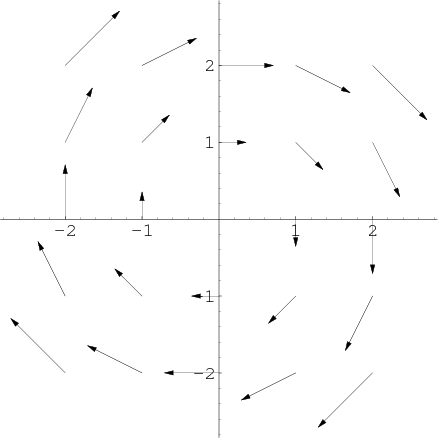# Math Insight

### Image: A 2D circulating vector fieldThis 2D vector field illustrates circulation. It is the vector field $\dlvf(x,y)=(y,-x)$.

Image file: vector_field_circulate.png